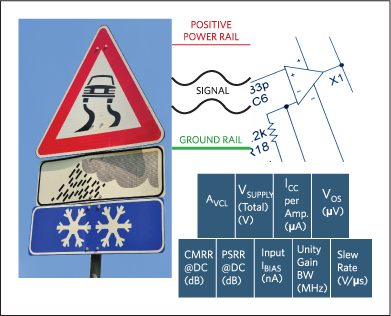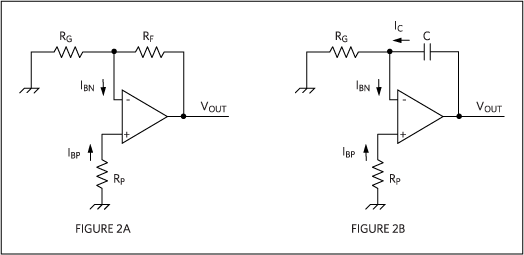# DC Error Characteristics of an Op Amp and the Effect on High-precision Applications

### Abstract

This article discusses the DC limitations of operational amplifiers and their effects, including input bias currents, input offset voltage, CMRR, PSRR, and input impedance. The article will provide the reader with a better understanding of how these limitations can create accuracy issues in high-precision applications.

A similar version of this article was published January 2014 in EDN.

### Introduction

Operational amplifiers, or op amps, are two-port integrated circuits (ICs) that apply precise gain on the external input signal and provide an amplified output as: input × closed-loop gain. Precision op amps behave close to ideal when operated at low to moderate frequencies and moderate DC gains. However, even under these conditions, op-amp performance is influenced by other factors that can impact accuracy and limit performance. Most common among these limitations are input referred errors that predominate in high-DC gain applications.

In this article we discuss the effects of input referred errors on op amps. These errors include input bias current, input offset current, input offset voltage, CMRR, PSRR, and finite input impedance. In reality, all these errors will occur at the same time. We also explain why a designer should be wary that the op-amp performance specifications described in the EC Table of a data sheet are only guaranteed for the conditions defined at the top of that table, unless otherwise noted as a specific characteristic. In reality, the effects of these DC errors change when the supply voltage, common-mode voltage range, and other conditions change.

### Errors Caused by Input Bias and Input Offset Currents

1

We are all familiar with potential dangers around us, and we engineers tend to forget that there are also dangerous traps to avoid when designing. Let’s see how this affects op amps (Figure 1A and 1B).IB = (IBP + IBN)/2 …..

IOS = IBP - IBN …..

where:

IB is average input bias current flowing into input pins;

IBP is input bias current flowing into the positive input;

IBN is input bias current flowing into the negative input;

IOS is the input offset current.

Input bias and input offset currents are two of the most critical characteristics in many precision amplifier applications; they affect the output with resistive and capacitive feedback. Many of the inverting, noninverting, summing, and differential amplifiers reduce to Figures 2A and 2B once their active inputs are set to zero. For this analysis, we set all input signals as zero to assess the effect of input currents on the output accuracy. We will analyze resistive feedback (Figure 2A) and capacitive feedback (Figure 2B) circuits separately.Applying the superposition theorem on Figure 2A yields:

VOUT = (1 + RF/RG) × [(RF//RG) × IBN – RP × IBP] ……

The following inferences can be made from Equation 3:

• Without any input signal, the circuit yields a finite output voltage. This unwanted output error is also called output DC noise.
• Output voltage is produced by amplifying the input error or input DC noise by (1 + RF//RG).
• Input DC noise has two components: voltage drop as IBP flows through RP, and voltage drop because IBN flows through a combination of RF//RG.

Depending on the level of precision needed in the application, we must make some careful choices for both passive component values and the op amp itself. This is the best way to nullify the effect of input bias current on output accuracy. Therefore, selecting RP = RF//RG yields:

VOUT = - (1 + RF/RG) × (RF//RG) × IOS …..

Selecting RP = RF//RG helps us reduce the output error in order of magnitude. But for high-precision applications where sensor interfaces are made with large gain (> 100V/V), it is still preferable to select low-input-offset-current op amps. Also, it is not always feasible to add RP. Finally, both input bias currents and resistance sizing play important roles in output error. For these situations designers should select op amps with low input-bias current, low input-offset voltage, a low speed-to-power ratio, and high CMRR and PSRR, such as the MAX44260, MAX9620, and MAX4238.

Output error can be further reduced by choosing lower RF and RG which, in turn, increase the circuit’s power dissipation. A careful trade-off between output error and power dissipation needs to be maintained when choosing the size of resistances.

We return now to Figure 2B. Voltages on both positive and negative inputs produce:

VIN+ = VIN- = -RP × IBP

where VIN+ is the voltage at the noninverting input, and VIN- is the voltage at the inverting input.

Applying Kirchhoff’s current law on inverting input yields:

VIN-/RG + IBN - IC = 0…..

We eliminate VIN- in Equation 6 by substituting Equation 5, which yields Equation 7 for input bias currents and current through a feedback capacitor:

IC = (RG × IBN - RP × IBP)/RG …..

Now apply Michael Faraday’s capacitance law:

VC = 1/C ƒIC dt

where VC is voltage across the capacitor, which is also VOUT. Substituting Equation 7 into Equation 8 yields:

VOUT = 1/(RG × C) × Integral(RG × IBN - RP × IBP)dt…..

Equation 9 provides the output voltage error in Figure 2B. To minimize this error, one can select RP = RG, and that reduces Equation 9 to:

VOUT = -1/(C) × Integral(IOS) dt …..

Since C and IOS are relatively constant, integrating Equation 10 over time would yield:

VOUT = -IOS × t/C …….

Equation 11 implies a voltage ramp that drives the op amp into saturation.

### Errors Caused by VOS and TCVOS

1

We will now explain the effect of input offset voltage on both the typical resistive and capacitive feedback in op-amp circuits.From Figure 3A, the output voltage error is:

VOUT = (1 + RF/RG) × VOS …..

where (1 + RF/RG) is DC noise gain. The bigger the resistances, the larger is the error.

From Figure 3B, we have IC = IRG for op amps with negligible input bias current; for VIN- = VOS, we have IC = IRG = VOS/RG. Using Faraday’s capacitance law yields:

VOUT = Integral(VOS) dt/(RG × C) …..

Again, if we integrate Equation 13 over time, the op-amp output is saturated to either rail depending on the polarity of the VOS.

An important conclusion can be made from Equations 12 and 13: for given values of passive resistances and capacitances, the offset voltage is the main contributor to the accumulated output-voltage error.

It is time for an example. Thermal drift of offset voltage (TCVos) and input offset voltage play a very critical role in precision applications where temperature variation is common. To emphasize the significance of TCVOS for an op amp in precision applications, we compare a typical op amp (maximum TCVOS = 5µV/°C and maximum VOS = 50µV) with the MAX9620 (maximum TCVOS = 0.12µV/°C and maximum VOS = 10µV). We can say that:

Maximum VOS(T) = max VOS(+25°C) + maximum TCVOS × (T-25°C)

Now we can use the MAX9620 op amp as an example. Assume that in a given application the temperature goes from room temperature (+25°C) to +125°C and that the maximum VOS due to thermal drift is:

Maximum VOS(T) = 10µV + 0.12µV/°C × (100°C) = 22µV ….

In contrast, an op amp with a 50µV maximum offset and 5µV/°C maximum TCVOS yields:

Maximum VOS(T) = 50µV + 5µV/°C × (100°C) = 550µV ….

These results show the importance of thermal drift for input offset voltage where high accuracy in applications is desired.2

### Errors Caused by CMRR and PSRR Limitations

Finite common-mode rejection ratio (CMRR) in typical op amps degrades precision by introducing an offset voltage at the input. The higher the CMRR of the amplifier, the more insensitive it is to input offset-voltage change over the rated input common-mode voltage. In applications where the input signal is very small, i.e., in the order of mV ranges, high CMRR is absolutely critical.

The CMRR of an amplifier is the ratio of differential gain (ADIFF) to common-mode gain (ACM). CMRR can also be expressed in terms of the change in the input offset voltage with respect to change in the input common-mode voltage (VCM) by 1V. Therefore:

Equation 17 can also be termed as:

VOUT = ADIFF × (VIN+ - VIN-) + ACM × VCM ……………….

Also:

CMRR = ADIFF/ ACM = delta (VCM)/delta(VOS)

Finite power-supply rejection ratio (PSRR) also plays an important role in introducing additional input offset voltage with respect to change in the power-supply voltage. A change in the power-supply voltage (VCC) alters the operating points of internal transistors which, in turn, affects the input offset voltage. The higher the PSRR, the more insensitive the amplifier will be to the change in input offset voltage when the power-supply voltage is changed.

PSRR = delta (VCC)/delta (VOS)

The CMRR and PSRR specs provided in the Electrical Characteristics (EC) table of an amplifier data sheet are specified at a particular input common-mode voltage and power-supply voltage range, respectively, unless otherwise noted. The CMRR spec provided is not the same over the entire power-supply range, and the PSRR spec provided is not the same over the entire input common-mode range.3

### Errors Caused by Input Impedance Limitations

Finite input impedance (RIN) of an op amp will form a voltage-divider with the source impedance (RS) driving the amplifier and introducing gain error. Consequently, a very high input impedance on the order of tens of 109 ohms is required to ensure negligible error.

In the above situation the amount of input signal (VIN) that the amplifier sees from a source depends on the input impedance parameter defined as:

VIN = VSOURCE × [RIN/(RIN+RS)]………………………..

From Equation 18 if RIN >>> RS, then VIN = VS.

### Summary

In conclusion, if DC errors like input offset voltage, input bias currents, and finite input impedance are not addressed, op-amp measurements will simply not be accurate. That performance is not acceptable in high-precision applications where accuracy is paramount. It is also essential that designers understand the significance and limitations of the op-amp performance specs defined in data-sheet EC tables. Following the guidelines presented here, designers can select both the correct op amp and the right passive components with the correct configurations for their applications. Ultimately, using the best op amp for a design will eliminate op-amp errors and ensure the highest accuracy possible.

### 参考电路

1. Sergio Franco, Design with Operational Amplifiers and Analog Integrated Circuits, Third Edition.
2. The MAX44250 and MAX4238 families of amplifiers also provide maximum input offset voltages in the order of 6µV and 2µV, respectively, which are needed for high-precision mV signal-level amplification in weigh scale and other sensor front-end applications.
3. The MAX44246, MAX44250, and MAX9620 families of amplifiers provide CMRR of 158dB, 140dB, and 135dB, respectively, and PSRR of 166dB, 145dB, and 135dB, respectively. Very high values of CMRR and PSRR are crucial in applications where high-precision DC performance is desired.